## Knot detection and classification

Knots in chromosome are detected based on the method established before to study protein chains  with optimizations taking into account the specific properties of chromosomes. Below, we summarize the most important information and modifications to the previous algorithm (http://knotprot.cent.uw.edu.pl/knot_detection)  concerning knot detection in chromosomes.

## Basic principles of knot detection on open chains

Knots are the basic objects studied in the mathematical field of knot theory. Knot theory studies entanglements in closed curves, although these ideas can be extended to characterize knotting in open chains [1,2,3,4,5]. To characterize knots in chromosomes, we follow the method established by us for proteins .

Knots are defined uniquely on closed chains only. To define them in open chains (which have loose ends), such as chromosomes, one must choose how to connect the two loose ends in order to close the chain. Making this choice in the most optimal way is the first difficulty we have to overcome when analyzing chromosomes; various ways how to form a close loop on proteins are discussed in . The methods used by KnotGenom are described here. Once this problem is addressed and an open chain is effectively transformed into a closed chain, we detect a knot type by computing polynomial knot invariants known as Alexander and HOMFLY polynomials.

A knot can be defined by the minimal number of crossings when projected onto a plane. Thus, the simplest nontrivial knot is called trefoil (denoted also by 31). A knot with four crossings is called figure-8 (denoted by 41), knots with five crossings are denoted by 51 and 52. There are three knots with six crossings, one of them is called Stevedore's knot (denoted by 61). An unknotted loop is called the trivial knot, or the unknot, and is denoted by 01. In the notation above, the first number denotes the minimal number of crossings a given knot can show in a projection (e.g. minimal number of crossings in a projection of a trefoil onto a plane is 3). The 31, 52 and 61 knots are chiral, i.e. they differ from their mirror images, and their complete characterization requires the determination of their chirality, which we denote by a plus (+) or a minus (-) sign next to the symbol of a knot. The 41 knot is an example of an achiral knot, i.e. it is identical to its mirror image and it cannot have assigned chirality.

Knots detected in chromosomes are much more complex than those presented above; moreover, multiple knots can be identified on a single chain, and one knot can be embedded in another one. Details about knot classification used in KnotGenom are described in Knot Classification section.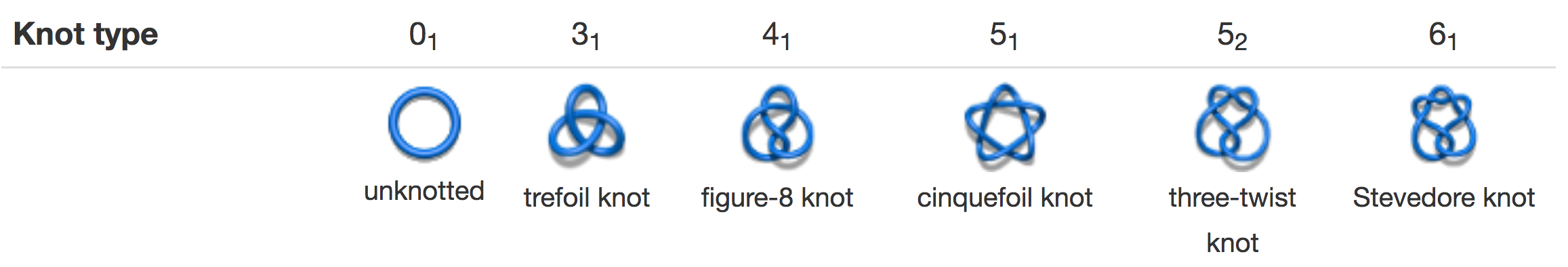Fig. 1 Examples of prime knots.Fig. 2 Examples of composite knot 31#31. Left panel — Granny knot, right panel — square knot.

## Methods to close the chromosome

It is impossible to unambiguously define the concept of knotting for an open chain . Only in the case of a closed chain the knot type is uniquely determined, and the techniques for classifying the knotting in open chains rely on closing the chain. However, for open chains the knot type can change depending on the way the chain is closed . To detect knots in a long single chromosome chain we used following methods:

• Random closure method (Fig. 3A). The chromosome endpoints are connected a certain number of times to two points randomly chosen on a large sphere. Subsequently, these two points are connected by an arc lying on the surface of the sphere. The most frequently observed knot type is then associated with that chain as its dominant knot type. As a default option, 20 closures are calculated. To increase probability of correct knot detection, the user can increase the number of random points to one hundred.
• The centre of mass method (Fig 3B). The chromosome endpoints are connected to two points on the sphere based on directions determined by their positions and the centre of mass. These two points are connected by an arc lying on the surface of the sphere.
• Direct closure method (Fig 3C), which connects chromosome endpoints by the shortest path.

Remark: In the case of a single chromosome, we recommended to use "the centre of mass" method.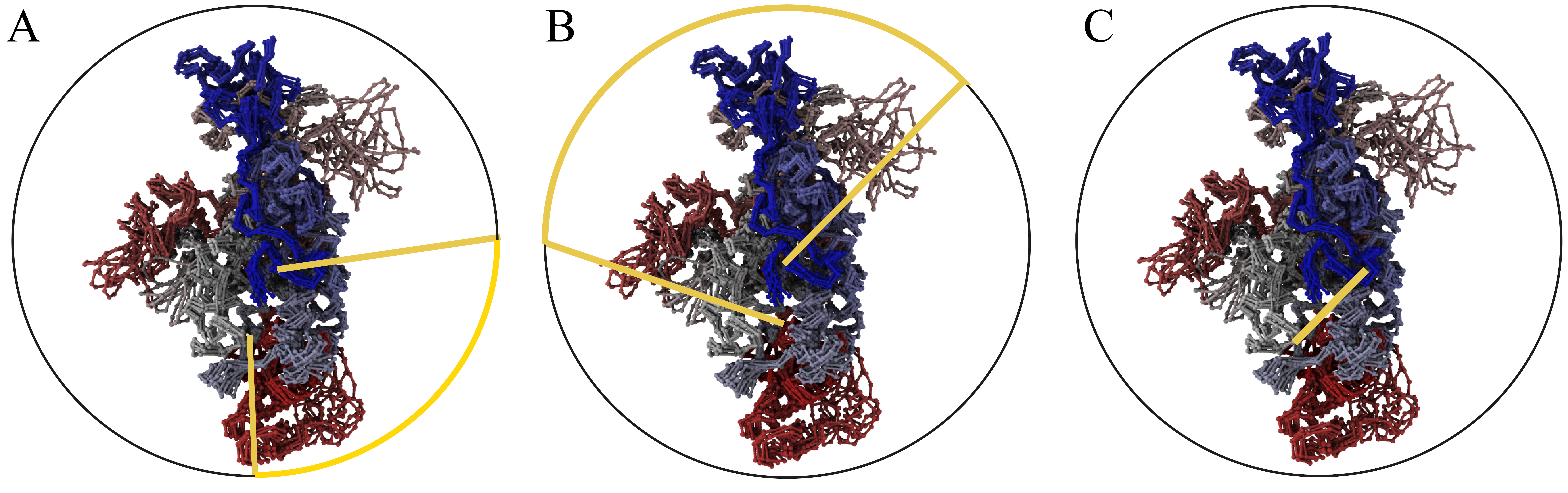Fig. 3 Schematic representation of a chromosome enclosed within a large sphere with different enclosure methods used in KnotGenom. Panel A — random closure method. Panel B — the centre of mass method. Panel C — direct closure method. The enclosing sphere is represented by a black circle, and an enclosing chain fragment is depicted with the yellow line.

## The knot type detection

The knot types resulting from individual closures are determined by computing polynomial knot invariants. For quick computations of all analyzed subchains, we use the Alexander polynomial. To detect chirality of formed knots, the HOMFLY polynomial is calculated for some of the analyzed subchains.

## Complexity reduction of chromosomes using the KMT algorithm

Computing knot polynomials is relatively fast for short chains, however, it can be a very time consuming process for long chains, and chromosomes are an order of magnitude longer than a typical protein (150 amino acids, each amino acid is represented as a single bead). Thus, to compute polynomials for a given chromosome, we used a method tested before for proteins. Before computing the Alexander polynomial for a given chain (or fixed subchain), after closing terminals (for each random closure separately), we first reduce it to a shorter configuration using the KMT algorithm [2,3]. This algorithm analyzes all triangles in a chain made by three consecutive beads, and removes the middle bead if no other segment of the chain intersects with the corresponding triangle. In effect, after a number of iterations, the initial chain is replaced by a (much) shorter chain of the same topological type.

## Knot classification

The algorithm used by the KnotGenom distinguishes knots up to 10 crossings (prime knots) and up to 12 composite knots. Currently knots with more crossings are called Other. Note, that the composite knot composed of "61 # 71" is called "Other". A prime knot is a knot which cannot be decomposed into a sum of two or more simpler knots. Composite knots are knot sums of prime knots, for instance 31 # 31 denotes a knot made of two trefoil knots, see Fig. 2,4-6. In the projection of a composite knot one can draw a line which divides it into two parts, each of which contains an independent knot.Fig. 4 Examples of composite knots found in the chromosome chains: 31 # 31 and 31 # 41.

In proteins we have found only prime knots. In contrast, our analysis of available data  indicates that composite knots are quite frequent in chromosomes. Below, a few examples of composite knots identified in chromosomes are presented.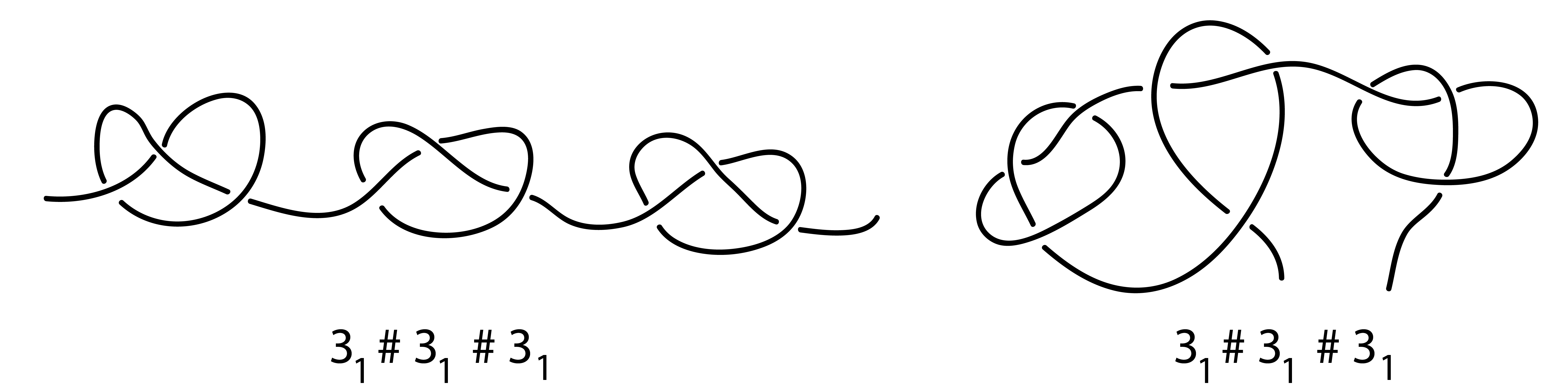Fig. 5 Examples of different types of composite knots 31 # 31 # 31 . Left panel: three 31 prime knots, are placed separable. Right panel: three 31 prime knots, are not placed separable along the chain.Fig. 6 An example of a complex composite knot found along the chromosome chain using KnotGenom.

## Knot table – example of classification and figures assignment

 Link name Image UnknotTrefoil +31+ and - denotes chirality.The same figures are used in both cases.Figure eight 41+51-51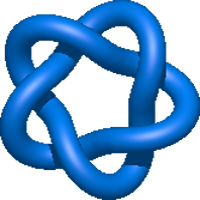... OtherOther denotes knots with more than 10 crossings.+31 # +31 +31 # -31 -31 # -31 -31 # +31 # denotes any composite knot. For example 31 # 31 denotes a knot made of two trefoil knots.The same figure is used for a sum of knots with different chirality.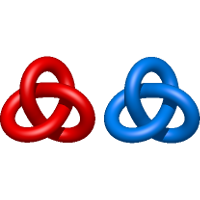... +31 # 41 41 # +31 The same figure is used for the sum of two composite knots composed of the same type of knot.The knots are ordered from the most complex to the simplex one31 # 31 # 31 Composite knots composed of more than 3 prime knots are represented with a graphic. No figureSuch figure is too complex to be well visualized. +31 # Other Example of a composite knot, sum of trefoil knot and knot with more than 10 crossings.Table 1. Knots - examples of classification and figures assignment.

 Sulkowska, J.I., Rawdon, E.J., Millett, K.C., Onuchic, J.N. and Stasiak, A. (2012) Conservation of complex knotting and slipknotting patterns in proteins. Proc. Natl. Acad. Sci. U.S.A. 109, E1715-E1723.
 M Jamroz, W Niemyska, EJ Rawdon, A Stasiak, KC Millett, P Sulkowski, JI Sulkowska, KnotProt: a database of proteins with knots and slipknots, Nucleic Acids Res. (2014), 43: D306-D314.
 Koniaris K, Muthukumar M (1991) Self-entanglement in ring polymers. J Chem Phys 95:2873-2881.
 Taylor WR (2000) A deeply knotted protein structure and how it might fold. Nature 406:916-919.
 Millett, K.C., Rawdon, E.J., Stasiak, A. and Sulkowska, J.I. (2012) Identifying knots in proteins. Biochem. Soc. Trans. 41, 533-537.

KnotGenom | Interdisciplinary Laboratory of Biological Systems Modelling